# Analogy

Analogy means similarity of relationship. If a pair of figures exhibits some kind of relationship on the basis of shape, size, rotation, interchange of elements, number of elements etc., and another pair of figures exhibits the same kind of relationship between them, then the two pairs are said to be analogous to each other.

Different types of questions covered in this section are as follows

1. Choosing One Element of a Similarly Related Pair

2. Finding the Odd Pair of Figures

3. Finding a Pair of Similar Figures in Pattern to Original Pair

4. Finding the Pair of Figures having Pattern other than the Original Pair

5. Selecting the Correct Figure on the Basis of Analogous Figure

6. Selecting the Correct Set of Figures to Establish Analogy

This section is all about identifying such logical patterns that establish a relationship between two given figures.

The basis for establishing such relationship between the different figures is given below:

1. Size of Figures

In this type of questions, the size of the figures in the first figure of one pair are interchanged to obtain the second figure of the pair, i.e., the smaller figure becomes larger and the larger figure becomes smaller. Similar relationship/pattern is followed to obtain the missing figure of another pair.

Example 1: Find the figure from the answer figure that will replace the question mark (?) from the problem figure.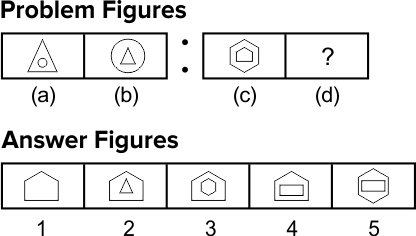Solution:  (3) By the careful analysis of first part of the problem figure, we find that from to second figure, the larger triangle become small and the small circle become large. In the same manner, from third to fourth figure, the large hexagon becomes small and the small pentagon become large as shown in figure (3).

2. Rotation of Figure

In this type of questions, first figure of one pair rotates by a certain angle to obtain the second figure of the pair. By following the same rotation pattern, the missing figure of another pair is to be found out.

Example 2: Find the figure from the answer figure that will replace the question mark (?) from the problem figure.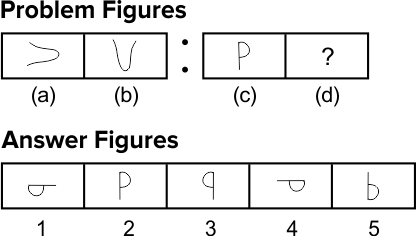Solution:  (4) By the careful analysis of first part of the problem figure, we find that first figure is rotated 900 clockwise to obtain the second figure. In the same manner, the third figure is rotated 900 clockwise to obtain the answer figure as given in option (4).

3. Number of Lines / Elements

In this type of questions, the number of lines get increased or decreased in the first figure of one pair to obtain the second figure of the pair. By following the same pattern , the missing figure of another pair is to be found out.

Example 3: Find the figure from the answer figure that will replace the question mark (?) from the problem figure.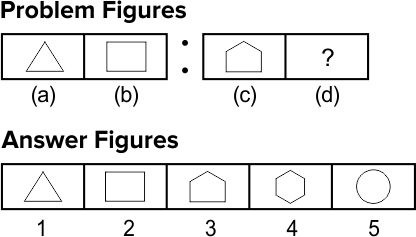Solution:  (4) By the careful analysis of first part of the problem figure, we find that first figure has three lines which become four in the second figure, i.e., form first to second figure one line is added. In the same manner, third figure has five lines which will transform into a six line figure in the fourth figure as shown in option (4).

4. Interchanging Position of the Figures

In this type of questions, the figures inside the first figure of one pair interchange their places to obtain the second figure of the pair. By following the same pattern, the missing figure of another pair is to be fount out.

Example 4: Find the figure from the answer figure that will replace the question mark (?) from the problem figure.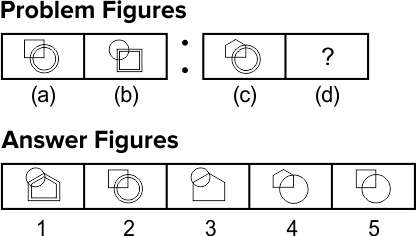Solution:  (1) By the careful analysis of first part of the problem figure, we find that first to second figure, the upper and lower figures interchange their places, i.e., the upper figure becomes lower and the lower figure becomes upper figure. The upper figure also appears in pair after interchange of position. Similarly, from third to fourth figure the upper and lower figures interchange places and also the interchanged figures appear in pair as shown in figure (1).

5. Rearrangement of Symbols

In this type of questions, the symbols of the first figure of one pair interchange their places with each other following a certain rule with or without producing a new symbol to obtain the second figure of the pair. By following the same rule, the missing figure of another pair is to be found out.

Example 5: Find the figure from the answer figure that will replace the question mark (?) from the problem figure.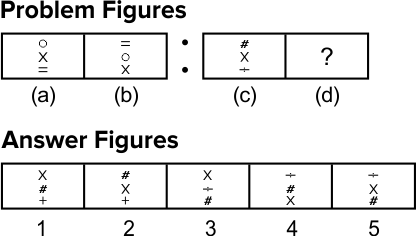Solution:  (4) By the careful analysis of first part of the problem figure, we find that first to second figure, each symbol moves one step downwards and the lower most symbol become the topmost symbol. Similarly from third to fourth figure, each symbol moves one step downward and the lowermost symbol become the topmost symbol as given in option (4).

Choosing One Element of a Similarly Related Pair

In this type of questions, two sets of figure namely problem figures and answer figures are given. The set of problem figures consists of two parts. The first part comprises of two figures which have some relationship between them on the basis of certain rule. The second part comprises one figure and a sign of '?' .

To solve this type of questions, candidates are required to select one figure from the set of answer figures which replaces the sign of '?' . In this case always remember that it bears same relationship with the other figure as first figure of the first part bears with second figure of the same part.

Following example illustrate types and methods to solve the questions on analogy

Directions (Example Nos. 6-7) In this following questions, the first and second figure of the problem figures have certain relation between them and the same relation exists between the third figure and one of the five answer figures (1), (2), (3), (4) and (5). Find that answer figure from the options that will replace '?' from problem figures.

Example 6: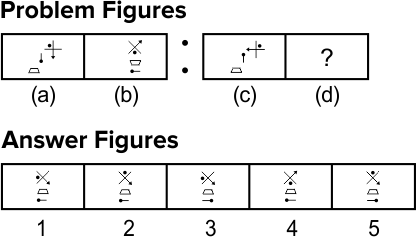Solution:  (3) From problem, figure (a) to (b), the pin rotates 900 clockwise, the trapezium is inverted and the third figure rotates 1350 anti-clockwise. The same rule will apply to obtain figure (d) from figure (c).

Example 7: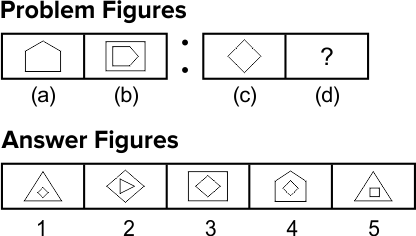Solution:  (1) From problem, figure (a) to (b), the pentagon rotates 900 clockwise, and a square (having four sides) covers it. Similarly, from figure (c) to (d) the square (having four sides) rotates 900 clockwise and will be covered by a triangle (having three sides).